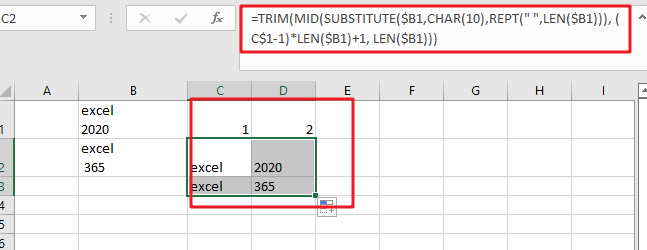# Extract Multiple Lines From A Cell

Suppose that you have listed some text in a single cell which is separated by the line break(you can do it by pressing `ALT + ENTER` after entering the text), and now you want to extract multiple lines of text from a single cell into a separate cell, just like it has been done in the screenshot below:

You would now think that you can do it manually, but keep it into your consideration that it seems easy when there are one or two cells from which you want to extract multiple lines of text into the separate cells, but when it comes to multiple cells from which you need to extract multiple lines of text into the separate cells then doing it manually would be the foolish attempt because by this you would get tired of it and would never complete your work on time.

But don’t be worry about it because after carefully reading this article, extracting multiple lines of text into separate cells would become a piece of cake for you.

So let’s dive into the article to take you out of this fix.

## General Formula:

The Following formula would help you out for extracting multiple lines of text into seprate cells:

`=TRIM(MID(SUBSTITUTE(B1,CHAR(10),REPT(” “,LEN(B1))), (N-1)*LEN(B1)+1, LEN(B1)))`

or

`=TRIM(MID(SUBSTITUTE(\$B1,CHAR(10),REPT(" ",LEN(\$B1))), (C\$1-1)*LEN(\$B1)+1, LEN(\$B1)))`## Syntax Explanations:

Before going into the explanation of the formula for getting the work done efficiently, we must understand each syntax which would make it easy for you that how each syntax contributes to extracting the multiple lines of text into the separate cells:

• `TRIM`: This function contributes to removing the extra spaces from the text, whether the space would be at the start or at the end of the text string.
• `REPT`: In MS Excel, the REPT function is used to repeat characters to a given number of times.
• `MID`: The MID function contributes to extracting the number (beginning from the left side) or characters from the given string.
• `FIND`: In MS Excel, the MID function contributes to finding out the specific text string inside the other.
• `LEN`: In MS Excel, the LEN functioncontributes to finding out the length of the text string.
• `SUBSTITUTE`: This function replaces the existing text with new text in a text string when you want to replace the text based on its content, not on its position.
• `B1`: It contributes to representing the input value.
• `Comma symbol (,)`: This comma symbol acts as a separator that separates the list of values.
• `Parenthesis ()`: The primary purpose of this parenthesis symbol is to group the various elements.
• `Minus Operator (-)`: This minus symbol contributes to subtracting any two values.
• `Plus operator (+)`: This plus symbol adds the values.

## Let’s See How This Formula Works:

The main point is that using the SUBSTITUTE and REPT functions, this formula searches for a line delimiter (“delim”) and then replaces it with many spaces.

Note that if you are using Excel on Mac and if your Excel version is old, then instead of using “CHAR(10)”, you should use “CHAR(13)” as for returning a character based upon its numeric code CHAR function is used.

The overall length of the text in the cell determines the number of spaces needed to replace the line delimiter. The formula then uses the MID function to extract the desired line. The following snippet of the formula carries out the starting point

(N-1)*LEN(B1)+1

In the above snippet, the “N” stands for “nth line,” which is picked up with the reference from the 1th row. The snippet “ LEN(B1) “ is used to identify the total number of characters extracted and is definitely equal to the length of the overall text string.

Now, to trim out all the extra space characters and to return just the line text, the TRIM function is used.

## Built-In Text to Columns Feature:

Moreover, you should know about it that there is also a built-in feature in MS Excel named Text to Columns feature (achieved by pressing Control + J ) that splits up the text according to the delimiter of your choice as if you are thinking to use this built-in way for extract multiple lines of text from a single cell into the separate cell instead of the formula explained above then stop here, as this built-in feature is not appreciated over the formula by the professionals because with the use of this built-in feature results are not more concise or desirable than the results after using the formula.

### Related Functions

• Excel TRIM function
The Excel TRIM function removes all spaces from text string except for single spaces between words. The syntax of the TRIM function is as below:=TRIM(text)…
• Excel MID function
The Excel MID function returns a substring from a text string at the position that you specify.The syntax of the MID function is as below:= MID (text, start_num, num_chars)…
• Excel Substitute function
The Excel SUBSTITUTE function replaces a new text string for an old text string in a text string. The syntax of the SUBSTITUTE function is as below:= SUBSTITUTE  (text, old_text, new_text,[instance_num])….
• Excel CHAR function
The Excel CHAR function returns the character specified by a number (ASCII Value).The CHAR function is a build-in function in Microsoft Excel and it is categorized as a Text Function. The syntax of the CHAR function is as below:=CHAR(number)….
• Excel REPT function
The Excel REPT function repeats a text string a specified number of times.The REPT function is a build-in function in Microsoft Excel and it is categorized as a Text Function.The syntax of the REPT function is as below:= REPT  (text, number_times)….
• Excel LEN function
The Excel LEN function returns the length of a text string (the number of characters in a text string).The LEN function is a build-in function in Microsoft Excel and it is categorized as a Text Function.The syntax of the LEN function is as below:= LEN(text)…